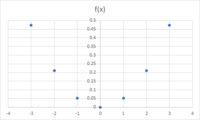# How do I graph these Quadratic functions?

#### Chart21

##### New member
1. f(x) = -1/19x2

Not sure what to do. If this was f(x) = 5x2 I'd do (0,0) as the vertex and make a table to figure out some other points. But I'm really stuck with this super odd fraction. A video told me to use a number that, when squared, is divisible by the fractions denominator. 19 squared is 361! I can't even graph that on my homework. I've also tried using a table and some fractions, but the rise/run fraction works when you don't have an x value already. I'm just really stuck here.

2. f(x) = 2(x-11)2

I know that the vertex is (11, 0). How do I calculate the y value?

Graphing f(x) = a(x-h)^2 is super hard for me. I've looked everywhere online and no one explains it. When I try solving it by using FOIL to turn it into general form, it's wrong. So obviously there's a way to solve these that isn't using general form.

#### Subhotosh Khan

##### Super Moderator
Staff member
1. f(x) = -1/19x2

Not sure what to do. If this was f(x) = 5x2 I'd do (0,0) as the vertex and make a table to figure out some other points. But I'm really stuck with this super odd fraction. A video told me to use a number that, when squared, is divisible by the fractions denominator. 19 squared is 361! I can't even graph that on my homework. I've also tried using a table and some fractions, but the rise/run fraction works when you don't have an x value already. I'm just really stuck here.

2. f(x) = 2(x-11)2

I know that the vertex is (11, 0). How do I calculate the y value?

Graphing f(x) = a(x-h)^2 is super hard for me. I've looked everywhere online and no one explains it. When I try solving it by using FOIL to turn it into general form, it's wrong. So obviously there's a way to solve these that isn't using general form.
I think you have an "aversion" towards plotting decimals. Get over it !! Just scale the y-axis appropriately and plot. The problem (1) will look as follows:

 x​ f(x)​ 0​ 0​ -1​ 0.052632​ 1​ 0.052632​ -2​ 0.210526​ 2​ 0.210526​ -3​ 0.473684​ 3​ 0.473684​Now show us your work for the second problem! For that problem - I would choose x = 11, 10, 12, 9, 13 (symmetrical about the vertex x = 11)

#### Chart21

##### New member
Our teacher said she specifically doesn't want to teach us how to graph with decimals because it's too complicated. I guess I'll go find tutorials on how to do that and try that way. The "get over it" was really unnecessary and rude. I literally don't know how to graph with decimals,it's not me being lazy. Thanks for explaining what you have so far.

#### lex

##### Full Member
1. Draw the graph of y=$$\displaystyle -x^2$$ and when you've done it, write $$\displaystyle \frac{ }{19}$$ under every number on your y-axis.
2. Just make a table and e.g. when x=3 do (3-11), square it, then multiply by 2
3. You need to draw two separate graphs for when a>0 e.g. draw something that looks like $$\displaystyle y=x^2$$, and when a<0, something like $$\displaystyle y=-x^2$$.
Work out where $$\displaystyle y=a(x-h)^2$$ will have its vertex and where it will cut on the y-axis and label those 2 points.

•Subhotosh Khan

#### Chart21

##### New member
Ohhh that makes sense. When you put it that way, i get it. Thanks

#### lex

##### Full Member
Good luck with it.